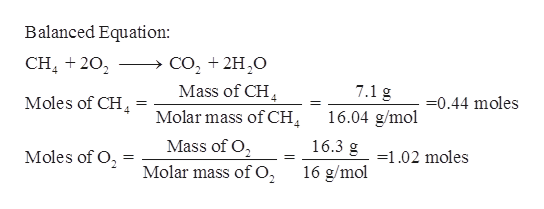# Gaseous methane CH4 will react with gaseous oxygen O2 to produce gaseous carbon dioxide CO2 and gaseous water H2O. Suppose 7.1 g of methane is mixed with 16.3 g of oxygen. Calculate the maximum mass of water that could be produced by the chemical reaction. Be sure your answer has the correct number of significant digits.

Question

Gaseous methane CH4 will react with gaseous oxygen O2 to produce gaseous carbon dioxide CO2 and gaseous water H2O. Suppose 7.1 g of methane is mixed with 16.3 g of oxygen. Calculate the maximum mass of water that could be produced by the chemical reaction. Be sure your answer has the correct number of significant digits.

check_circleExpert Solution
Step 1

Given,

Mass of methane = 7.1 g

Mass of oxygen = 16.3 g

First write balanced equation for the given reaction.  Calculate moles of reactants and then determine the limiting reactant as follows.help_outlineImage TranscriptioncloseBalanced Equation: со, + 2H,0 Mass of CH Molar mass of CH CH 202 4 7.1 g -0.44 moles Moles of CH 16.04 g/mol 4 16.3 g 102 moles Mass of O Moles of O = Molar mass of O, 16 g/mol fullscreen
Step 2

Determination of limiting reactant:

From the balanced equation of reaction, it is known that one mole of methane reacts with two moles of oxygen.

So, 0.44 moles of methane react with 2*0.44 = 0.88 moles of oxygen.

From ...

### Want to see the full answer?

See Solution

#### Want to see this answer and more?

Solutions are written by subject experts who are available 24/7. Questions are typically answered within 1 hour*

See Solution
*Response times may vary by subject and question
Tagged in

### Chemistry